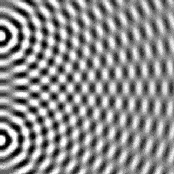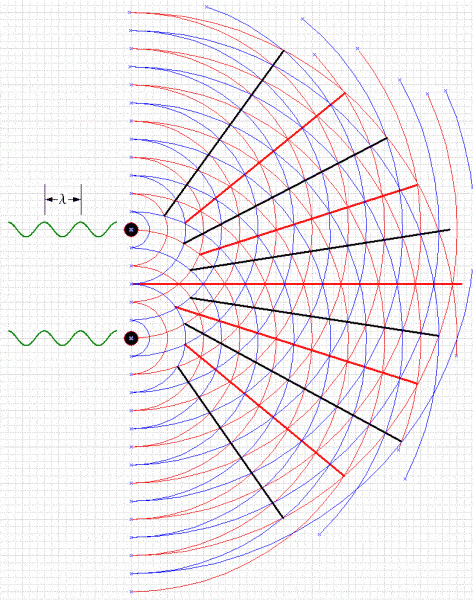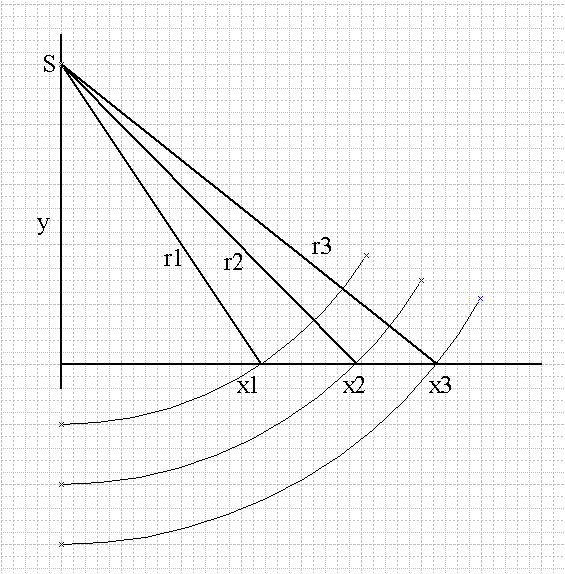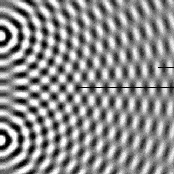# Measuring Wavelength Homework: Middle of Lines or Book?

• EgpYo
In summary, the book says that the wavelength is 1 cm and the picture looks like this, it has two slits. The Attempt at a Solution thinks that the wavelength is 0.5 cm and when he measures from the middle of a line to the middle of the next, he gets 0.5 cm. Um. How are we to judge the scale of the image? Does the book state that it is "actual size", or do they provide some reference measurement like the distance between the wave sources? The question just says to measure it, like with a ruler.f

## Homework Statement

Im not sure how to measure the wavelength when given this picture.

## Homework Equations

The picture looks like this, it has two slits.## The Attempt at a Solution

My book says that the wavelength is 1 cm. When I measure from the middle of a white line to the middle of the next white line, I get 0.5cm. Same for the black lines. Am I missing something here or isn't that how youre supposed to find the wavelength? Just want to determine if its me or the book that's wrong.

Um. How are we to judge the scale of the image? Does the book state that it is "actual size", or do they provide some reference measurement like the distance between the wave sources? Note that "actual size" won't help too much here, since the image size will depend upon browser settings and the like.

The question just says to measure it, like with a ruler. I got 0.5cm, but the answers section says 1cm. I am basically asking, is this the correct WAY to measure? If I measure from the middle of a line to the middle of the next, does this represent the wavelength? Or am I misunderstanding how these diagrams work?

I find this unit really confusing. Is wavelength even held constant throughout the entire diagram? If I draw lines for PS1 and PS2 at different locations (along nodal lines or antinodal lines) and do the wavelength calculations, should I get different wavelengths?

I believe that you are doing it correctly, measuring from peak-to-peak (or trough-to-trough). You want to measure wavelength along a radial line from a source. A horizontal line extending from one of the slits would be convenient.

What I don't understand is how wavelength seems to change. What do they mean by "measure it"?
For example, if I choose a point along the central antinodal line. The distance from PS1 and PS2 will be the same and m = 0

Lets say Ps1 and PS2 = 5cm.
|5-5| = 0 lambda
0 wavelength??

Lets say I am measuring a point along the first antinodal line from the center, m =1.
PS1 = 5.5cm and PS2 = 5cm
5.5-5 = 1lambda
wavelength = 0.5 cm

And it changes from nodal line to nodal line and from antinodal line to antinodal line. Is this correct?

I don't get it, whenever I measure and calculate wavelength along a nodal or antinodal line, I always get a different wavelength. Is this normal?

You could measure the distance for multiple wavelengths, then divide to arrive at one wavelength. That way measurement uncertainties tend to diminish.

I don't get it, whenever I measure and calculate wavelength along a nodal or antinodal line, I always get a different wavelength. Is this normal?
Measure radially outwards from a source, not at some angle to this.

I don't get it, whenever I measure and calculate wavelength along a nodal or antinodal line, I always get a different wavelength. Is this normal?
I would expect there to be a geometrical "projection" effect that alters the measured distance depending upon the angle of the line of measure with respect to a radius from a point source. The effect should diminish as you go further from the sources (so the wave fronts meet at a smaller angle (along the central line of antinodes they will become progressively more "vertical" with distance from the sources).

Here's a big drawing of an interference pattern. The wave peaks are shown as red circles, the troughs as blue circles. Lines of destructive interference (lines of nodes) are in black. Lines of constructive interference are in red.As you can see, the waves from the sources are more parallel when they meet further away from the sources.

Here's a diagram that illustrates what I meant by the geometrical projection effect:Successive wave peaks from a source S positioned at distance y above the central horizontal line of constructive interference meet the line at x1, x2, x3, and so on. It should be clear that x2-x1 is longer than x3-x2, and that neither is exactly equal to one wavelength. In fact, one wavelength would be equal to r2-r1, or r3-r2. Or two wavelengths would be equal to r3-r1.

Distances r1, r2, etc., should be fairly easy to measure. Perhaps if you can count peaks along the line of antinodes and measure the radial distances to the ends you can get a good estimate of the wavelength.

I understand the first picture you posted (with the different colors representing peaks and troughs). And whenever I put the ruler to the screen, I can always calculate the wavelength to be the same along antinodal or nodal lines, wherever I measure. This is not the case with the black and white picture. I do not get how to read the black and white picture. Can the black lines represent peak meeting peak or trough meeting trough? Then wavelength would be double the distance between two black lines.

I still don't get why wavelength is different whenever I try to measure it in my textbook.

It says:
a) Measure the wavelength of the waves, the distance between the sources, and the path distance from each of the sources to point P. Show complete calculation for wavelength.
b) Choose any point on any antinodal line and show complete calculation for wavelength.

Should I get the same answer for a and b?

the distance between the sources, and the path distance from each of the sources to point P. Show complete calculation for wavelength.
I enlarged your photo and took measurements. I calculated λ as the path difference to first bright fringe off the central maximum (imagining a screen located along the right side of the photo). The result matches what I find with direct measurement between any two bright concentric circles.

So if as you say the book's answer is double this, then it seems the book must be wrong.Last edited:
That isn't the interference pattern. I just found one similar on the internet to show what it looks like. Its black and white instead of actually showing the waves like the picture gneill posted. But I need to know: Is the calculated wavelength for part a) supposed to be the same as part b)? Because I am not getting the same wavelength when I do measurements.

So here we are worrying about not the interference pattern. Great. Well, you did try to warn us ("looks like this")...

Could you do us all and yourself a favour and fill in the following:

## The Attempt at a Solution

please be as complete as possible.